# Test: Basic Laws- 2

## 15 Questions MCQ Test Topicwise Question Bank for Electrical Engineering | Test: Basic Laws- 2

Description
Attempt Test: Basic Laws- 2 | 15 questions in 45 minutes | Mock test for Electrical Engineering (EE) preparation | Free important questions MCQ to study Topicwise Question Bank for Electrical Engineering for Electrical Engineering (EE) Exam | Download free PDF with solutions
QUESTION: 1

### The current I in the circuit shown below is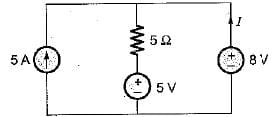Solution:

Let the current flowing through 5 Ω resistor be I' Applying KCL, we get
5 + I = I'.
Applying KVL, we get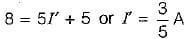So,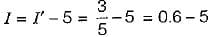= -4.4 A

QUESTION: 2

### For the circuit shown below, match List - I with List-II and select the correct answer using the codes given below the lists: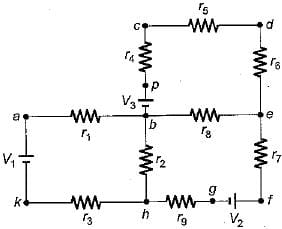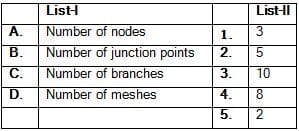Codes: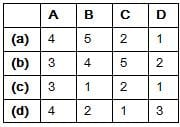Solution:

Nodes = 10 (a, b, c, d, e, f, g, h, k, p)
Junction points = 3(b, e, h)

QUESTION: 3

### The value of current I in the circuit shown below for V = 2 volt is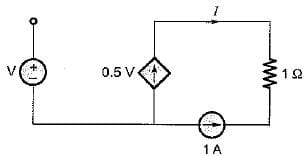Solution:

Applying KCL at the node connecting 0.5 V current source and 1 A current source, we get
1 + I = 0.5 V
For v - 2 volt, 1 + I = 1
or, I = 0 A

QUESTION: 4

For the circuit shown below, the value of (V1+ V2) is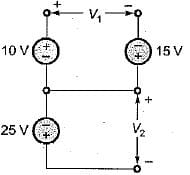Solution:

Using KVL in the loop, we have
V= 10 + 15 = 25 volts
Also, V2 = -25 volts
∴ V1 + V2 = 25 - 25 = 0 volts

QUESTION: 5

The value of voltage source to be connected across the terminals X and Y so that drop across the 10 Ω resistor is 45 V is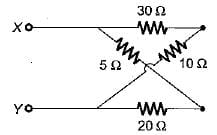Solution:

Let the required voltage be V
Then, voltage across 10 Ω resistor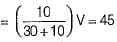or,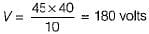Hence, required voltage is
V = 180 volts

QUESTION: 6

In a voltage divider circuit as shown below with two resistances R1 and R2, the voltage drop V1 is twice the drop V2 across R2. The value of R1, and R2 will be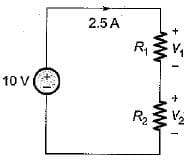Solution:

Using Ohm’s law,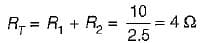Given, V1 = 2V2
As I is same therefore,
R1 = 2R2
∴ RT = R1 + R2 = 2R2 + R2 = 3R2
So, RT = 3R2 = 4Ω
or,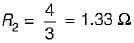and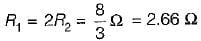QUESTION: 7

A delta connected load is shown in figure below. The equivalent star connection has a value of R in Ω is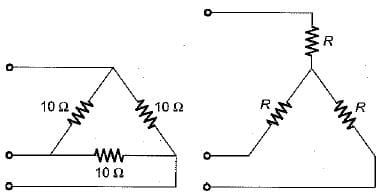Solution:

We know that
RΔ = 3 RY
or,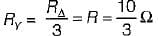QUESTION: 8

The voltage drop across the 2 Ω resistor for the circuit shown below is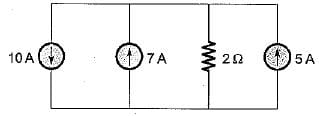Solution:

On combining the current sources, the circuit is reduced as shown below.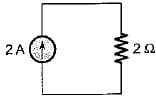Hence, voltage drop across the 2Ω resistor
= 2 x 2 = 4 volts

QUESTION: 9

For the circuit shown below, the sum of the unknown currents I1, I2, l3 and I4 is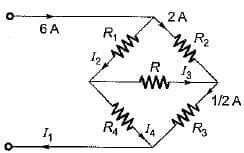Solution:

Using KCL,
I2 = 6 - 2 = 4 A
Also,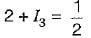or,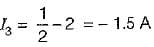and I4 = I- I3 = 4 - (-1.5) = 5.5 A
Also, I1 = 6 A
∴ I1 + I2 + I3 + I4 = 6 + 4 - 1.5 + 5.5
= 15.5 - 1.5 = 14A

QUESTION: 10

In the delta equivalent of the given star connected circuit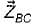is equal to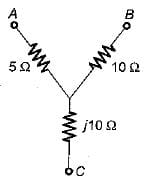Solution: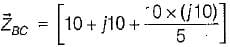= (10 + j10 + j20)
= (10 + j30) Ω

QUESTION: 11

Four resistances 80 Ω, 50 Ω, 25 Ω and R are connected in parallel. Current through 25 Ω resistance is 4 A. The total current of the supply is 10 A. The value of R will be

Solution: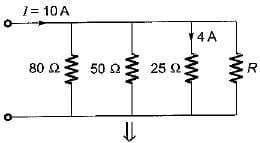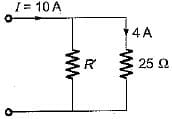Given, I25Ω=4 A
Here, R' = 80║50║R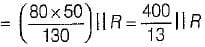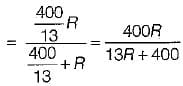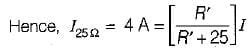or,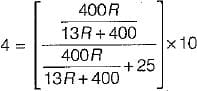or,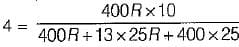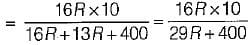or, 29R + 400 = 40R or 11R = 400
or, R = 36.36 Ω

QUESTION: 12

For the circuit shown below, the equivalent resistance will be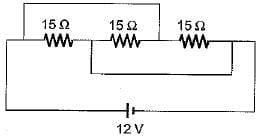Solution: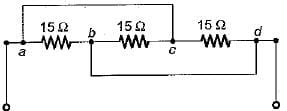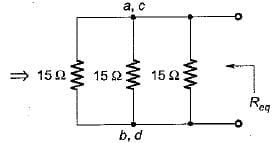Here, a and c are at equipotential.
Also, b and d are at equipotential
∴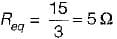QUESTION: 13

Three resistors of R Ω each are connected to form a triangle. The resistance between any two terminals will be

Solution: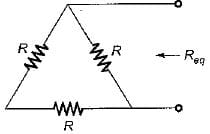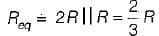QUESTION: 14

A network has 10 nodes and 17 branches. The number of different node pair voltage would be

Solution:

Number of different node-pair voltage = Number of KCL equations = n - 1 =10 - 1 = 9

QUESTION: 15

Kirchhoff's laws are not applicable to circuits with

Solution:Use Code STAYHOME200 and get INR 200 additional OFF Use Coupon Code